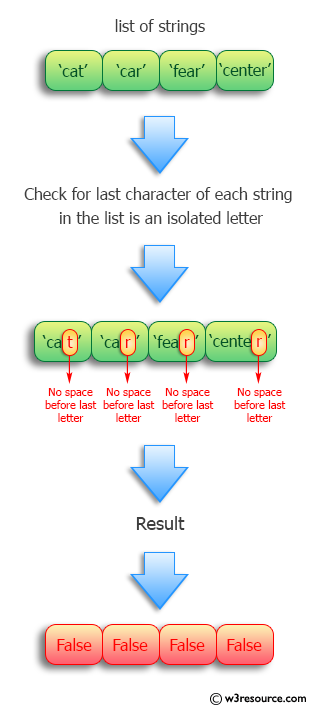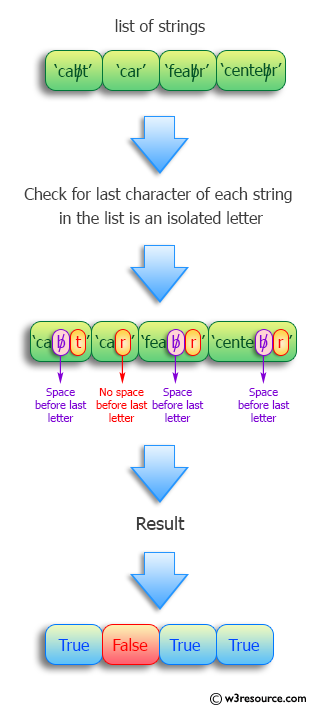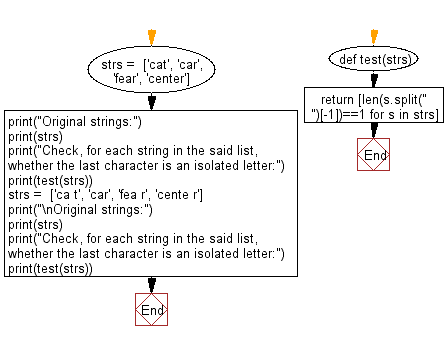﻿ Python: Determine, for each string in a list, whether the last character is an isolated letter - w3resource# Python: Determine, for each string in a list, whether the last character is an isolated letter

## Python Programming Puzzles: Exercise-21 with Solution

Write a Python program to check, for each string in a given list, whether the last character is an isolated letter or not. Return True or False.

```Input:
['cat', 'car', 'fear', 'center']
Output:
[False, False, False, False]

Input:
['ca t', 'car', 'fea r', 'cente r']
Output:
[True, False, True, True]
```

Pictorial Presentation:Sample Solution:

Python Code:

``````#License: https://bit.ly/3oLErEI

def test(strs):
return [len(s.split(" ")[-1])==1 for s in strs]
strs =  ['cat', 'car', 'fear', 'center']
print("Original strings:")
print(strs)
print("Check, for each string in the said list, whether the last character is an isolated letter:")
print(test(strs))
strs =  ['ca t', 'car', 'fea r', 'cente r']
print("\nOriginal strings:")
print(strs)
print("Check, for each string in the said list, whether the last character is an isolated letter:")
print(test(strs))
``````

Sample Output:

```Original strings:
['cat', 'car', 'fear', 'center']
Check, for each string in the said list, whether the last character is an isolated letter:
[False, False, False, False]

Original strings:
['ca t', 'car', 'fea r', 'cente r']
Check, for each string in the said list, whether the last character is an isolated letter:
[True, False, True, True]
```

Flowchart:## Visualize Python code execution:

The following tool visualize what the computer is doing step-by-step as it executes the said program:

Python Code Editor :

Have another way to solve this solution? Contribute your code (and comments) through Disqus.

What is the difficulty level of this exercise?

Test your Programming skills with w3resource's quiz.

﻿

## Python: Tips of the Day

Clamps num within the inclusive range specified by the boundary values x and y:

Example:

```def tips_clamp_num(num,x,y):
return max(min(num, max(x, y)), min(x, y))
print(tips_clamp_num(2, 4, 6))
print(tips_clamp_num(1, -1, -6))
```

Output:

```4
-1
```